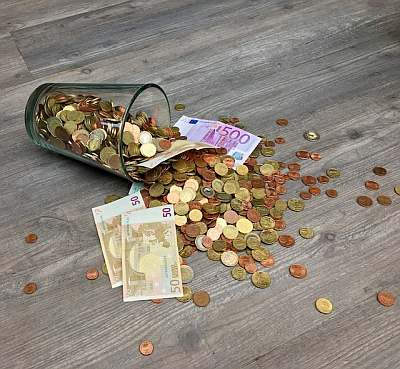# Payback Period Calculator

Instructions: Use this Payback Period Calculator to compute the Payback Period ($$PP$$) of a stream of cash flows by indicating the yearly cash flows ($$F_t$$), starting at year $$t = 0$$:Type the yearly cash flows (comma or space separated)

More about the Payback period calculator so you can better understand the way of using this calculator: The payback period of a stream of cash flows $$F_t$$ is number of years it takes a project to break even. Typically, projects require a cash outlay at the beginning ($$t = 0$$), and they typically receive positive cash inflows until the amount received equals the initial outlay. They time that takes is called payback period

### How do you calculate the payback period?

The methodology is simple: You need to compute the cumulative cash flows, and find the period where the cumulative cash flows first turn from negative to positive. Then, an interpolation is conducted to estimate the exact portion of year needed to reach a cumulative cash flow equal to zero.

### What type of measure is the payback period?

The payback period (or PBP) is a measure of profitability, that is often use to evaluate projects. Its main criticism is that it does not take into account the time value of money.

### What is simple payback and when do we use discounting?

Observe that this calculator uses the flows without discounting. In other words, you are computing a payback period without a discount rate. If you want to get use discounted cash flows, you should probably use our discounted payback period calculator , in which the methodology is exactly the same, only that in order to compute cumulative flows, the discounted cash flows are considered instead.

### Other finance calculators for project evaluation

In order to evaluate a project, you can also use this net present value (NPV) or this internal rate of return calculators.### Example of the calculation of the payback period

Question: You are the manager of a new project that requires $10,000 of upfront outlay. It is expected that the project will bring a revenue of$3,000 at the end of the first year, and \$4,000 at the end of the following 3 years. Compute the payback period of the project.

Solution:

This is the information we have been provided with:

• The cash flows provided are: -10000, 3000, 4000, 4000, 4000.

Finding the payback period corresponds to finding the number of years where the initial negative outlay is matched by positive cash inflows.

Based on the cash flows provided, the following table shows the corresponding cumulative cash flows:

 Period Cash Flows Cumulative Cash Flows 0 -10000 -10000 1 3000 -7000 2 4000 -3000 3 4000 1000 4 4000 5000

Observe that the cumulative cash flows at year $$t = 2$$ is negative, and the cumulative cash flows at year $$t = 3$$ is positive, so then the payback period is somewhere between two years. Using interpolation we get:

$\begin{array}{ccl} PBP & = & 2 + \displaystyle \left( \frac{3000}{1000 + 3000}\right)\times (3 - 2) \\\\ \\\\ & = & 2 + 0.75 \\\\ \\\\ & = & 2.75 \end{array}$

Therefore, for the provided cash flows, the payback period is $$PBP = 2.75$$ years.